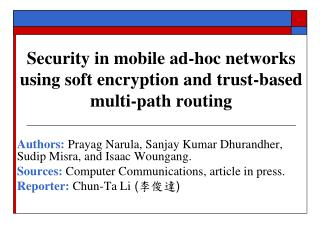# Security in mobile ad-hoc networks using soft encryption and trust-based multi-path routing - PowerPoint PPT PresentationDownload PresentationSecurity in mobile ad-hoc networks using soft encryption and trust-based multi-path routing

Security in mobile ad-hoc networks using soft encryption and trust-based multi-path routing
Download Presentation## Security in mobile ad-hoc networks using soft encryption and trust-based multi-path routing

- - - - - - - - - - - - - - - - - - - - - - - - - - - E N D - - - - - - - - - - - - - - - - - - - - - - - - - - -
##### Presentation Transcript

1. Security in mobile ad-hoc networks using soft encryption and trust-based multi-path routing Authors:Prayag Narula, Sanjay Kumar Dhurandher, Sudip Misra, and Isaac Woungang. Sources: Computer Communications, article in press. Reporter: Chun-Ta Li (李俊達)

2. Outline • Motivation • Proposed routing strategy • Comments 2 2

3. Motivation • Message security using trust-based multi-path routing in MANETs • Multi-path routing (on-demand routing) • divide the message into different parts • Soft encryption • eliminate the need of Key Distribution Center and key transfer • using the message itself for encryption • Trustworthiness • trusted node is allowed to feature in more paths

4. Proposed routing strategy • Trust level (-1 to 4) • 4 : complete trust • 0 : initial trust • -1: complete distrust • Multi-path routing track and listen neighboring nodes leave node destination source

5. Proposed routing strategy (cont.) • On-demand routing protocol A B C R_REQ E F G D S R_REP H I J The R_REP message is appended with the trust level of the successor node by the node

6. Proposed routing strategy (cont.) • Message encryption • A 4n-bits message is divided into four parts of n bits each (denote these parts by a, b, c and d) a’ = a XOR c b’ = b XOR d c’ = c XOR b d’ = d XOR a XOR b

7. Proposed routing strategy (cont.) • Message decryption • A 4n-bits message is divided into four parts of n bits each (denote these parts by a, b, c and d) a = b’ XOR d’ b = a’ XOR b’ XOR c’ XOR d’ c = a’ XOR b’ XOR d’ d = a’ XOR c’ XOR d’

8. Proposed routing strategy (cont.) • Trust defined strategy • 4 : those nodes can be given the right to read the full message • 3 : those nodes can be sure of finding 2n possible messages of which one would be correct • 2 : those nodes can be sure of finding 2n * 2n possible messages • 1 : those nodes can be sure of finding 2n * 2n * 2n possible messages

9. Proposed routing strategy (cont.) • Algorithm to select secure routes

10. Proposed routing strategy (cont.) • Example (four parts) a’ a’ 1 1 1 a’ a’ a’ 2 b’ 1 1 b’ c’ b’ c’ D S c’ b’ 1 3 b’ c’ c’ d’ 2 2 d’ d’ d’ 1 d’

11. Comments • A node with normal trust level may easily read the partial message and the property of confidentiality would not be achieved. c’ c’ 1 1 1 c’ d’ 2 c’ 1 1 d’ a’ c’ a’ d’ D S b’ 1 3 d’ d’ b’ b’ b’ 2 2 a’ {a, c} a’ b’ 1 a’ {a}

12. Comments (cont.) • A node with normal trust level may easily read the full message and the property of confidentiality would not be achieved. c’ b’ d’ {a, c} b’ a’ d’ a b’ XOR d’ c a c’ a’ c’ d’ d’ {d} XOR XOR c’ b c XOR a’ c’ b’ Ø d XOR b b’

13. Comments (cont.) • If a, b, c, and d are encrypted by means of the following equations a’ = a XOR b XOR c b’ = a XOR b XOR d c’ = a XOR c XOR d d’ = b XOR c XOR d {b} b’ a’ d’ a’ c’ d’ {c} a’ c’ b’ {a} c’ {d} b’ d’

14. Comments (cont.) • Fabrication attack • Lack of mutual authentication between source and destination node • Payment system  Nuglets (virtual currency) A B C R_REQ Nuglets (SB) Nuglets (SC) Nuglets (SA) E F G D S Nuglets (SE) Nuglets (SF) Nuglets (SG) R_REP Nuglets (SH) H I J Nuglets (SI) Nuglets (SJ)